Like   Tweet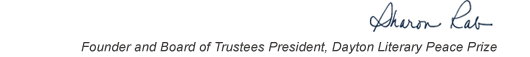table div table+table+table+table div table{width:100%;padding:0}table div table+table+table+table div table img{width:96.23%;padding:0;float:none}table div table+table+table+table div table td{width:100%;padding:0 1.88% 18px}/* styles */This list includes the winners and runners-up for 2006 and we have rich background information on each one on our website.

▪ Under Past Winners. scroll down to the year and click on it.
▪ If you click on the winners and runners-up, you will find the author’s bio, the judges’ citation, and the writer’s reflection on literature and peace.
 ▪ Under Past Winners. scroll down to the year and click on it.
 ▪ If you click on the winners and runners-up, you will find the author’s bio, the judges’ citation, and the writer’s reflection on literature and peace.

On the bottom right of the home page, you can click on COREScholar, which is built by the Chair of our Curriculum Committee Carol Loranger, Associate Dean of the College of Liberal Arts at Wright State, and maintained for us by Wright State University. There you can find interviews, TED Talks, articles, other books, films, etc. by and about each of our winning and runner-up authors. It is a great resource for students, book clubs, and readers who would like to explore an author in depth.

 table div table+table+table+table+table+table div table{width:100%;padding:0}table div table+table+table+table+table+table div table img{width:96.23%;padding:0;float:none}table div table+table+table+table+table+table div table td{width:100%;padding:0 1.88% 18px}/* styles *//* styles */ “Read global; buy local.” — Marlon James, DLPP 2009 Fiction Winner for The Book of Night Women
 table div table+table+table+table+table+table+table+table div table{width:100%;padding:0}table div table+table+table+table+table+table+table+table div table img{width:96.23%;padding:0;float:none}table div table+table+table+table+table+table+table+table div table td{width:100%;padding:0 1.88% 18px}/* styles */## 2006 Finalists - Fiction

 table div table+table+table+table+table+table+table+table+table+table div table{width:100%;padding:0}table div table+table+table+table+table+table+table+table+table+table div table img{width:96.23%;padding:0;float:none}table div table+table+table+table+table+table+table+table+table+table div table td{width:100%;padding:0 1.88% 18px}/* styles */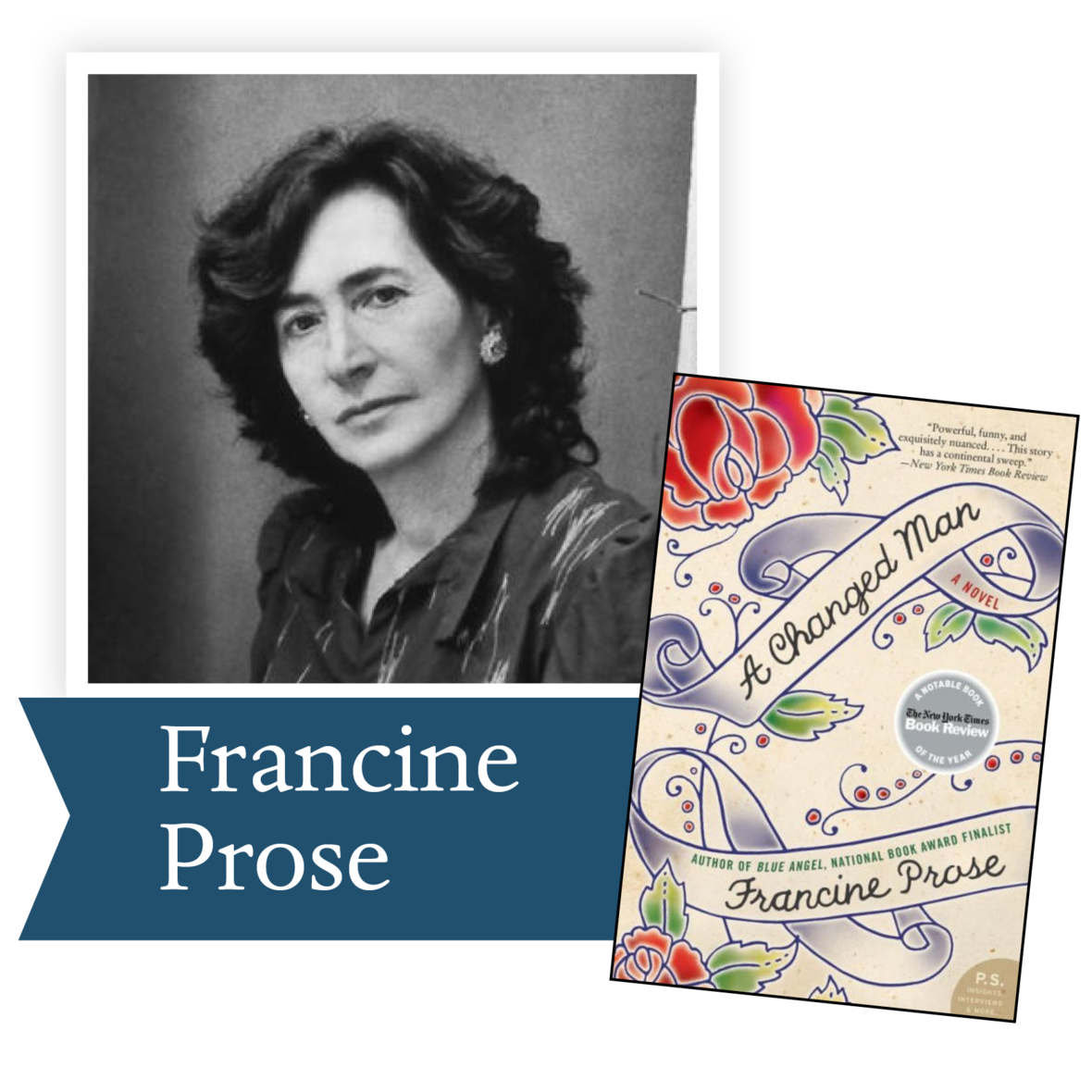A Changed Man by Francine Prose (HarperCollins): Francine Prose’s novel A Changed Man boldly portrays the psychological roots of social conflict in the character of Vincent Nolan, a young American neo-Nazi who takes a great leap of faith and attempts to transform his own life for the better.

 table div table+table+table+table+table+table+table+table+table+table+table+table div table{width:100%;padding:0}table div table+table+table+table+table+table+table+table+table+table+table+table div table img{width:96.23%;padding:0;float:none}table div table+table+table+table+table+table+table+table+table+table+table+table div table td{width:100%;padding:0 1.88% 18px}/* styles */In Perfect Light by Benjamin Alire Saenz (HarperCollins): From award-winning poet Benjamin Alire Sáenz comes In Perfect Light, a haunting novel depicting the cruelties of cultural displacement and the resilience of those who are left in its aftermath.

 table div table+table+table+table+table+table+table+table+table+table+table+table+table+table div table{width:100%;padding:0}table div table+table+table+table+table+table+table+table+table+table+table+table+table+table div table img{width:96.23%;padding:0;float:none}table div table+table+table+table+table+table+table+table+table+table+table+table+table+table div table td{width:100%;padding:0 1.88% 18px}/* styles */The Discontinuity of Small Things by Kevin Haworth (Quality Words in Print): This quiet story of the Holocaust chronicles the lives of Danes through the summer of 1943. The discontinuity of small things—the scattered inconveniences, chance meetings, glimpses of injustice, and indulgences of hope,— haphazardly direct individual fate. A hypnotic story of ordinary people caught in a silent maelstrom driven to extraordinary feats.

 table div table+table+table+table+table+table+table+table+table+table+table+table+table+table+table+table div table{width:100%;padding:0}table div table+table+table+table+table+table+table+table+table+table+table+table+table+table+table+table div table img{width:96.23%;padding:0;float:none}table div table+table+table+table+table+table+table+table+table+table+table+table+table+table+table+table div table td{width:100%;padding:0 1.88% 18px}/* styles */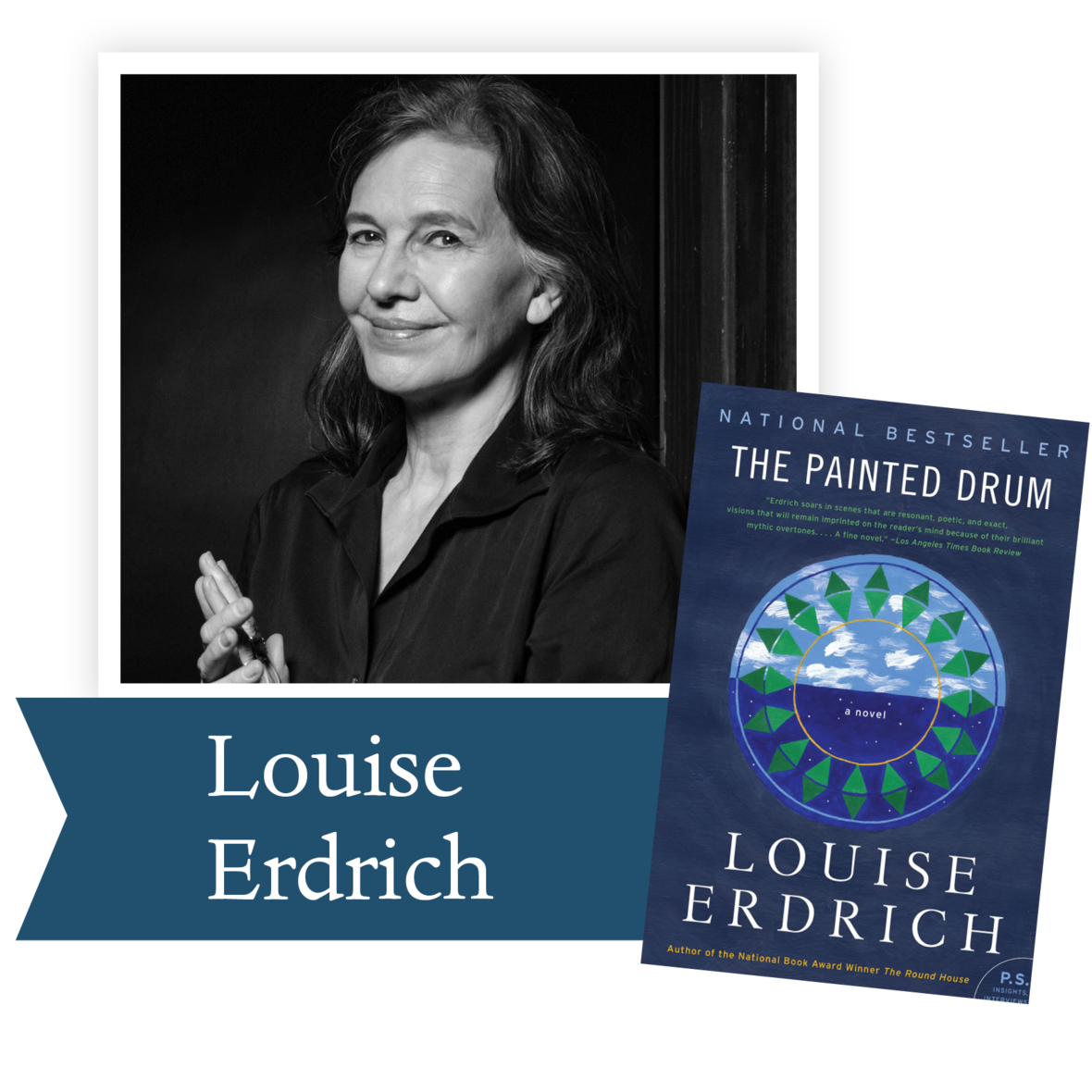The Painted Drum by Louise Erdrich (HarperCollins): Compelling and unforgettable, Louise Erdrich's Painted Drum explores the often-fraught relationship between mothers and daughters, the strength of family, and the intricate rhythms of grief with all the grace, wit, and startling beauty that characterizes this acclaimed author's finest work.

 table div table+table+table+table+table+table+table+table+table+table+table+table+table+table+table+table+table+table div table{width:100%;padding:0}table div table+table+table+table+table+table+table+table+table+table+table+table+table+table+table+table+table+table div table img{width:96.23%;padding:0;float:none}table div table+table+table+table+table+table+table+table+table+table+table+table+table+table+table+table+table+table div table td{width:100%;padding:0 1.88% 18px}/* styles */War by Candlelight by Daniel Alarcon (Harper Collins): Something is happening around the globe: mass movements of peoples, dislocations of language and culture in the wake of war and economic crises—simply put, our world is changing. In this exquisite collection, Daniel Alarcón takes the reader from Third World urban centers to the fault lines that divide nations and people.

 table div table+table+table+table+table+table+table+table+table+table+table+table+table+table+table+table+table+table+table+table div table{width:100%;padding:0}table div table+table+table+table+table+table+table+table+table+table+table+table+table+table+table+table+table+table+table+table div table img{width:96.23%;padding:0;float:none}table div table+table+table+table+table+table+table+table+table+table+table+table+table+table+table+table+table+table+table+table div table td{width:100%;padding:0 1.88% 18px}/* styles */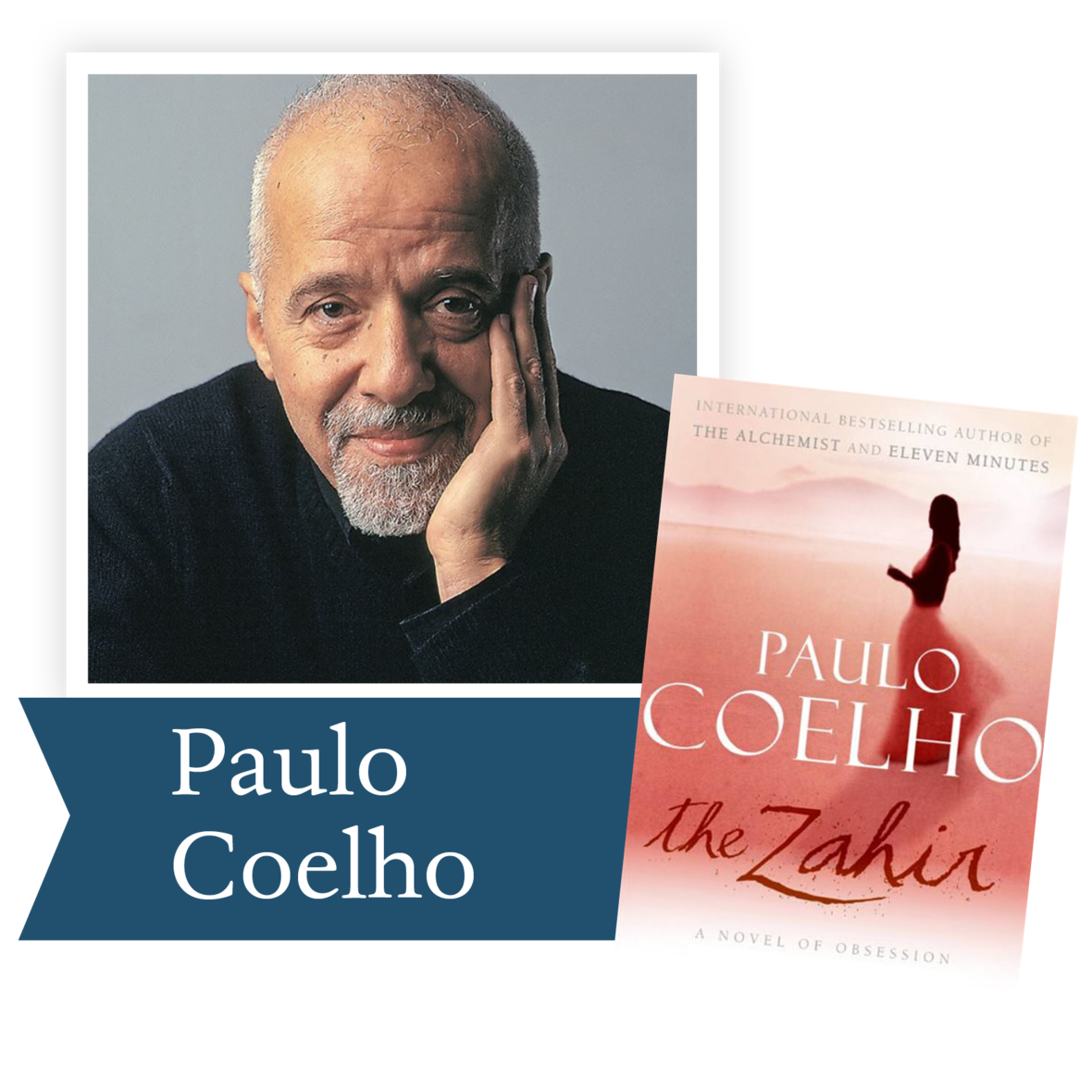Zahir: A Novel of Obsession by Paulo Coelho (HarperCollins): The narrator of The Zahir is a bestselling novelist who lives in Paris and enjoys all the privileges money and celebrity bring. His wife of ten years, Esther, is a war correspondent who has disappeared along with a friend, Mikhail, who may or may not be her lover.

Was Esther kidnapped, murdered, or did she simply escape a marriage that left her unfulfilled? The narrator doesn’t have any answers, but he has plenty of questions of his own. Then one day Mikhail finds the narrator and promises to reunite him with his wife. In his attempt to recapture a lost love, the narrator discovers something unexpected about himself.

 table div table+table+table+table+table+table+table+table+table+table+table+table+table+table+table+table+table+table+table+table+table+table div table{width:100%;padding:0}table div table+table+table+table+table+table+table+table+table+table+table+table+table+table+table+table+table+table+table+table+table+table div table img{width:96.23%;padding:0;float:none}table div table+table+table+table+table+table+table+table+table+table+table+table+table+table+table+table+table+table+table+table+table+table div table td{width:100%;padding:0 1.88% 18px}/* styles */## 2006 Finalists - Nonfiction

 table div table+table+table+table+table+table+table+table+table+table+table+table+table+table+table+table+table+table+table+table+table+table+table+table div table{width:100%;padding:0}table div table+table+table+table+table+table+table+table+table+table+table+table+table+table+table+table+table+table+table+table+table+table+table+table div table img{width:96.23%;padding:0;float:none}table div table+table+table+table+table+table+table+table+table+table+table+table+table+table+table+table+table+table+table+table+table+table+table+table div table td{width:100%;padding:0 1.88% 18px}/* styles */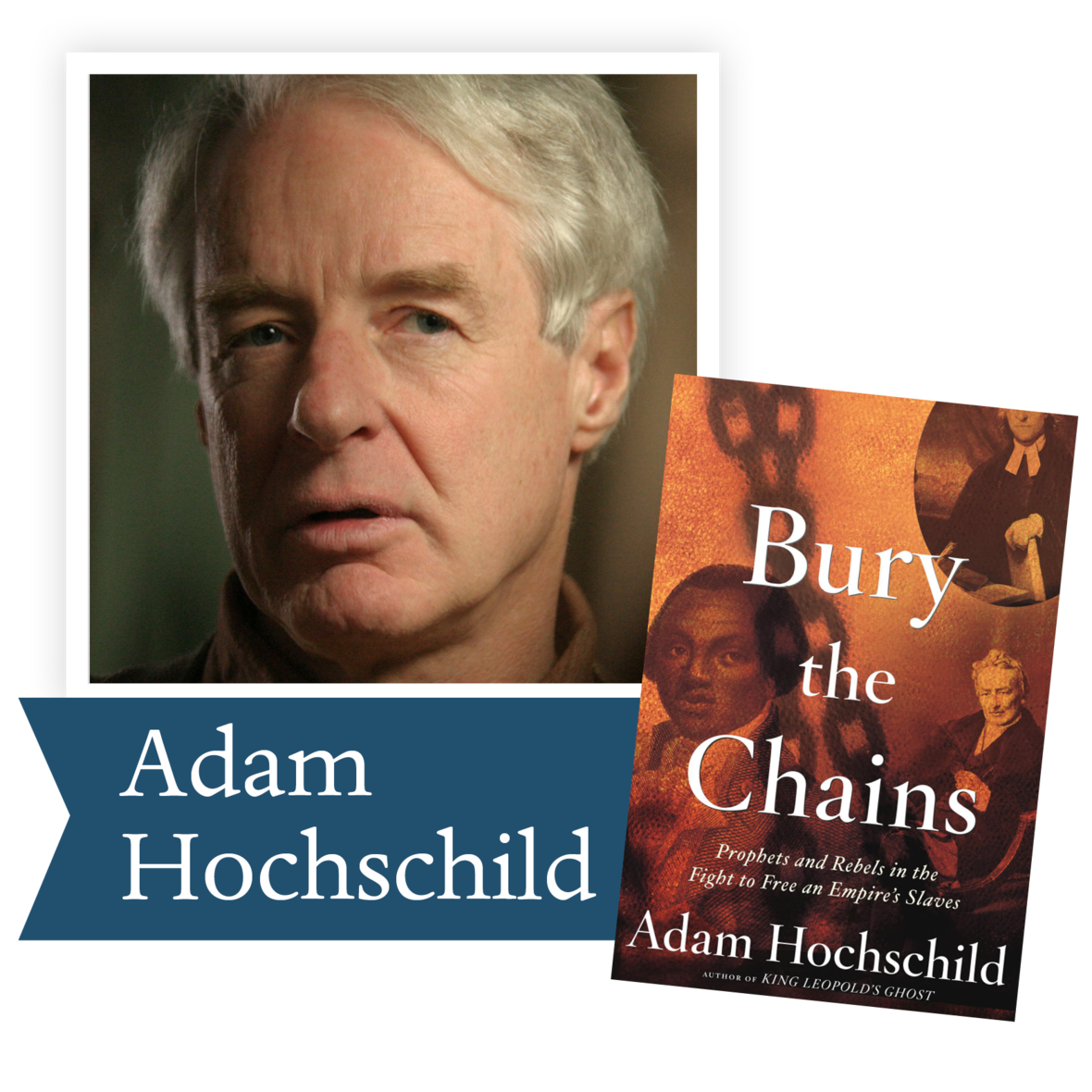Bury the Chains: Prophets and Rebels in the Fight to Free an Empire’s Slaves by Adam Hochschild (Houghton Mifflin): An account of the first great human rights crusade, which originated in England in the 1780s and resulted in the freeing of hundreds of thousands of slaves around the world.

 table div table+table+table+table+table+table+table+table+table+table+table+table+table+table+table+table+table+table+table+table+table+table+table+table+table+table div table{width:100%;padding:0}table div table+table+table+table+table+table+table+table+table+table+table+table+table+table+table+table+table+table+table+table+table+table+table+table+table+table div table img{width:96.23%;padding:0;float:none}table div table+table+table+table+table+table+table+table+table+table+table+table+table+table+table+table+table+table+table+table+table+table+table+table+table+table div table td{width:100%;padding:0 1.88% 18px}/* styles */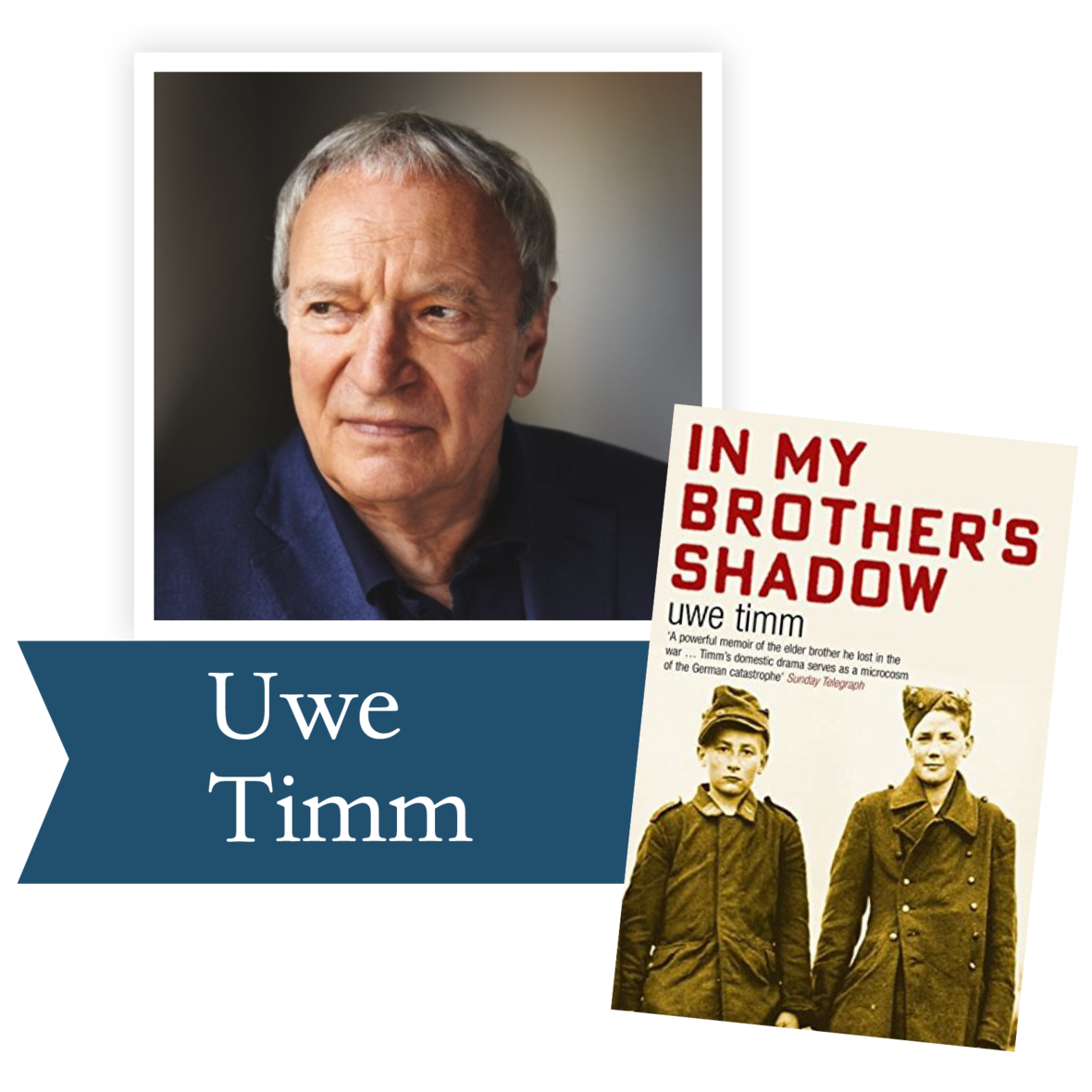In My Brother’s Shadow by Uwe Timm (Farrar, Straus and Giroux): Uwe Timm was born in Germany in 1940. Just three years later his brother, Karl-Heinz, who was sixteen years his senior and a sapper in the elite SS Death's Head Division, was killed. His notebook was returned to the family. When Timm decided to write this astonishing memoir, he feared the possibility that his brother's unit had taken part in the shooting of civilians and Jews. Yet he wanted to piece together his brother's experience, and also that of his nation, which once considered the qualities of an SS man so exemplary. As Timm unleashes his memories of this devastating time, he also pinpoints the questions that his parents' generation seemed unable to face, and offers new insights into the impact of the war on ordinary Germans.

 table div table+table+table+table+table+table+table+table+table+table+table+table+table+table+table+table+table+table+table+table+table+table+table+table+table+table+table+table div table{width:100%;padding:0}table div table+table+table+table+table+table+table+table+table+table+table+table+table+table+table+table+table+table+table+table+table+table+table+table+table+table+table+table div table img{width:96.23%;padding:0;float:none}table div table+table+table+table+table+table+table+table+table+table+table+table+table+table+table+table+table+table+table+table+table+table+table+table+table+table+table+table div table td{width:100%;padding:0 1.88% 18px}/* styles */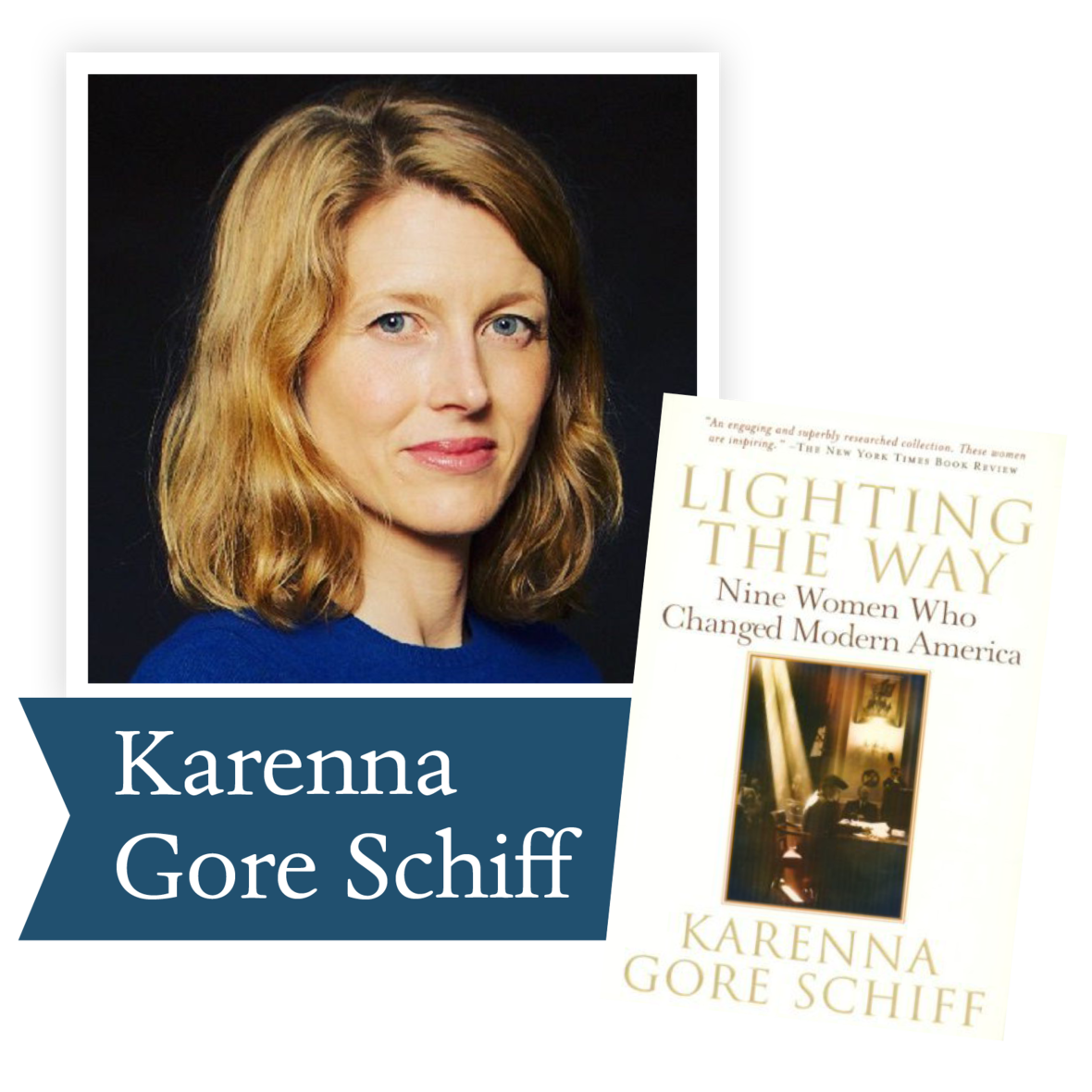Lighting the Way by Karena Gore Shiff (Miramax): Karenna Gore Schiff’s nationally bestselling narrative tells the fascinating stories of nine influential women, who each in her own way, tackled inequity and advocated change throughout the turbulent twentieth century.

 table div table+table+table+table+table+table+table+table+table+table+table+table+table+table+table+table+table+table+table+table+table+table+table+table+table+table+table+table+table+table div table{width:100%;padding:0}table div table+table+table+table+table+table+table+table+table+table+table+table+table+table+table+table+table+table+table+table+table+table+table+table+table+table+table+table+table+table div table img{width:96.23%;padding:0;float:none}table div table+table+table+table+table+table+table+table+table+table+table+table+table+table+table+table+table+table+table+table+table+table+table+table+table+table+table+table+table+table div table td{width:100%;padding:0 1.88% 18px}/* styles */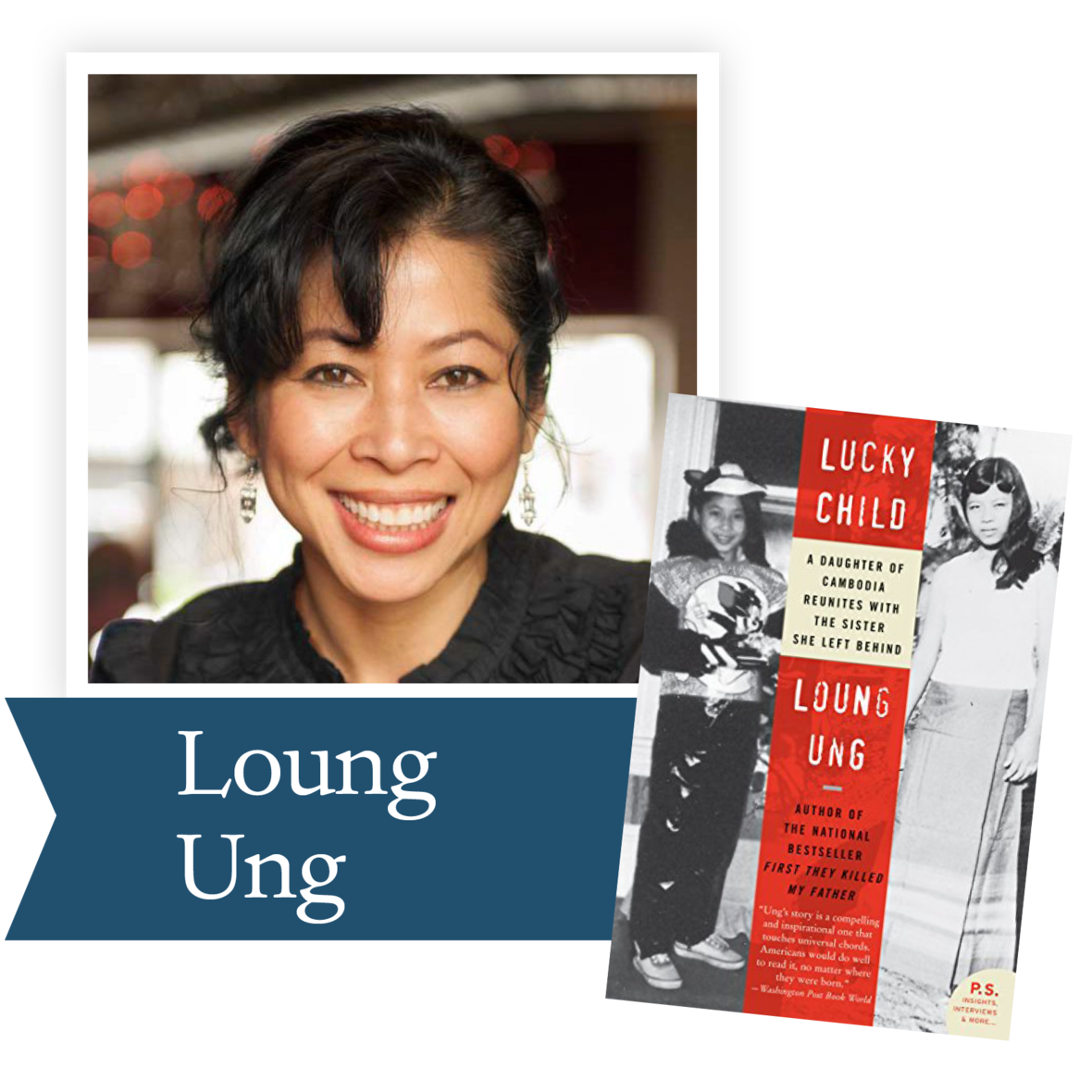Lucky Child by Loung Ung (Little, Brown and Company): In this poignant and elegiac memoir, Loung recalls her assimilation into an unfamiliar new culture while struggling to overcome dogged memories of violence and the deep scars of war. In alternating chapters, she gives voice to Chou, the beloved older sister whose life in war-torn Cambodia so easily could have been hers. Highlighting the harsh realities of chance and circumstance in times of war as well as in times of peace, Lucky Child is ultimately a testament to the resilience of the human spirit and to the salvaging strength of family bonds.

 table div table+table+table+table+table+table+table+table+table+table+table+table+table+table+table+table+table+table+table+table+table+table+table+table+table+table+table+table+table+table+table+table div table{width:100%;padding:0}table div table+table+table+table+table+table+table+table+table+table+table+table+table+table+table+table+table+table+table+table+table+table+table+table+table+table+table+table+table+table+table+table div table img{width:96.23%;padding:0;float:none}table div table+table+table+table+table+table+table+table+table+table+table+table+table+table+table+table+table+table+table+table+table+table+table+table+table+table+table+table+table+table+table+table div table td{width:100%;padding:0 1.88% 18px}/* styles */Night Draws Near by Anthony Shadid (Henry Holt and Company): Through the moving stories of individual Iraqis, Shadid shows how Saddam's downfall paved the way not just for hopes of democracy but also for the importation of jihad and the rise of a bloody insurgency.

 table div table+table+table+table+table+table+table+table+table+table+table+table+table+table+table+table+table+table+table+table+table+table+table+table+table+table+table+table+table+table+table+table+table+table div table{width:100%;padding:0}table div table+table+table+table+table+table+table+table+table+table+table+table+table+table+table+table+table+table+table+table+table+table+table+table+table+table+table+table+table+table+table+table+table+table div table img{width:96.23%;padding:0;float:none}table div table+table+table+table+table+table+table+table+table+table+table+table+table+table+table+table+table+table+table+table+table+table+table+table+table+table+table+table+table+table+table+table+table+table div table td{width:100%;padding:0 1.88% 18px}/* styles */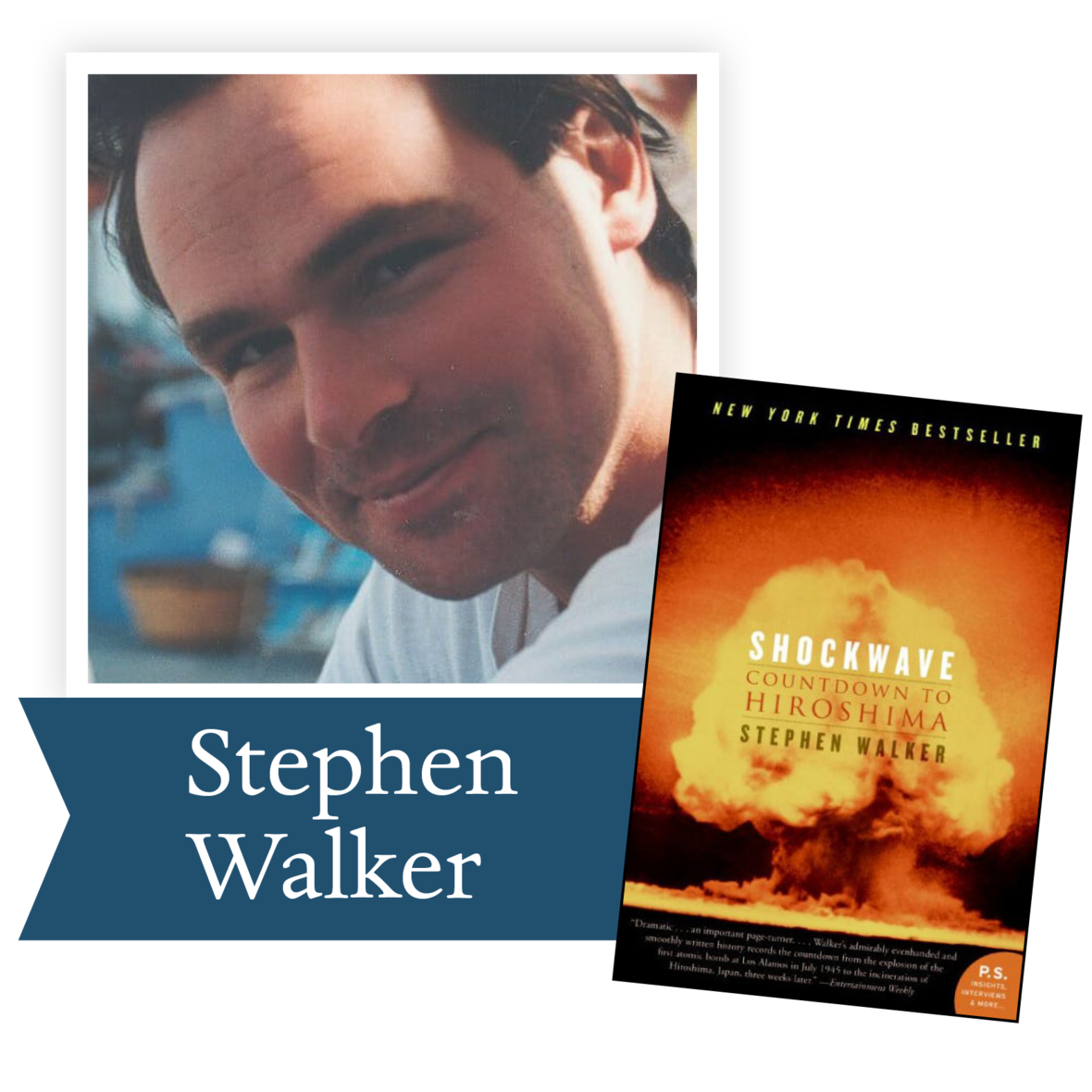Shockwave: Countdown to Hiroshima by Stephen Walker (HarperCollins): Author Stephen Walker brilliantly re-creates the three terrible weeks leading up to the wartime detonation of the atomic bomb—from the first successful test in the New Mexico desert to the cataclysm and its aftermath—presenting the story through the eyes of pilots, scientists, civilian victims, and world leaders who stood at the center of earth-shattering drama. It is a startling, moving, frightening, and remarkable portrait of an extraordinary event—a shockwave whose repercussions can be felt to this very day.

 table div table+table+table+table+table+table+table+table+table+table+table+table+table+table+table+table+table+table+table+table+table+table+table+table+table+table+table+table+table+table+table+table+table+table+table+table div table{width:100%;padding:0}table div table+table+table+table+table+table+table+table+table+table+table+table+table+table+table+table+table+table+table+table+table+table+table+table+table+table+table+table+table+table+table+table+table+table+table+table div table img{width:96.23%;padding:0;float:none}table div table+table+table+table+table+table+table+table+table+table+table+table+table+table+table+table+table+table+table+table+table+table+table+table+table+table+table+table+table+table+table+table+table+table+table+table div table td{width:100%;padding:0 1.88% 18px}/* styles */## 2006 Lifetime Achievement Award Winner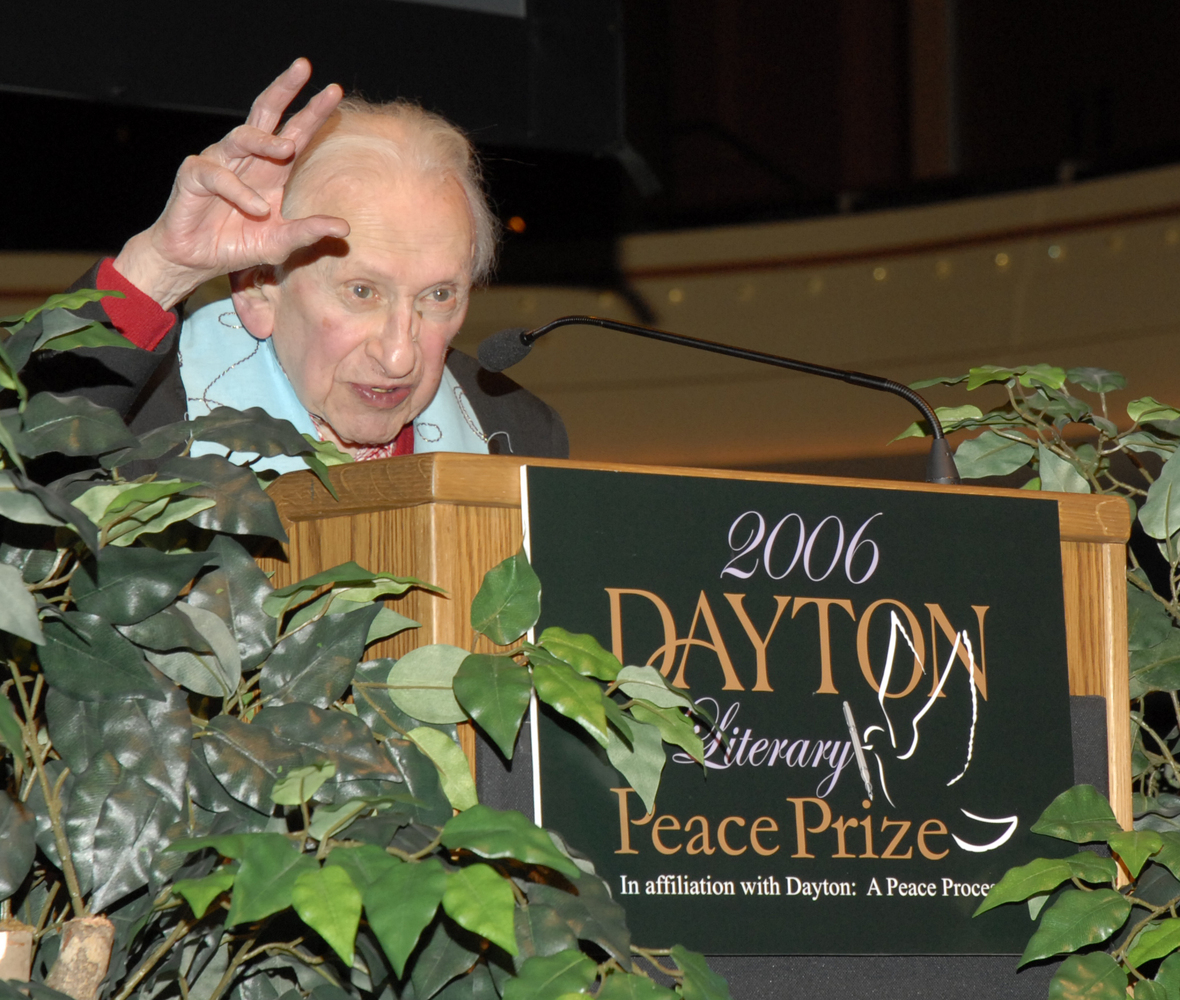In early 2006, WYSO’s Book Nook host Vick Mickunas suggested that we add a Lifetime Achievement Award to the fiction and nonfiction awards we had already planned. And then he suggested that we give it to Studs Terkel. We will be indebted forever to Vick for his idea and his willingness to invite Studs to Dayton for what Mr. Terkel called his “Valedictory Speech.” He was the perfect choice to launch the Dayton Literary Peace Prize, every bit the visual depiction of the common man he championed in his red shirt and red socks and with his crooked index finger admonishing us to “do what is right.”

We were honored to have him among us and we are honored to share his memory and his books with you. The list of books provides us with a rich oral history as, along with the taped archive of his radio show The Art of Conversation, he captures the voices of America. If you don’t know his work, his voice and the voices he captured on the page and on the air tell the true story of the country’s struggle toward greatness.table div table+table+table+table+table+table+table+table+table+table+table+table+table+table+table+table+table+table+table+table+table+table+table+table+table+table+table+table+table+table+table+table+table+table+table+table+table+table+table+table+table div table{width:100%;padding:0}table div table+table+table+table+table+table+table+table+table+table+table+table+table+table+table+table+table+table+table+table+table+table+table+table+table+table+table+table+table+table+table+table+table+table+table+table+table+table+table+table+table div table img{width:96.23%;padding:0;float:none}table div table+table+table+table+table+table+table+table+table+table+table+table+table+table+table+table+table+table+table+table+table+table+table+table+table+table+table+table+table+table+table+table+table+table+table+table+table+table+table+table+table div table td{width:100%;padding:0 1.88% 18px}/* styles */## Join us for a live stream panel discussion July 28 at 6:00 pm

 table div table+table+table+table+table+table+table+table+table+table+table+table+table+table+table+table+table+table+table+table+table+table+table+table+table+table+table+table+table+table+table+table+table+table+table+table+table+table+table+table+table+table+table div table{width:100%;padding:0}table div table+table+table+table+table+table+table+table+table+table+table+table+table+table+table+table+table+table+table+table+table+table+table+table+table+table+table+table+table+table+table+table+table+table+table+table+table+table+table+table+table+table+table div table img{width:96.23%;padding:0;float:none}table div table+table+table+table+table+table+table+table+table+table+table+table+table+table+table+table+table+table+table+table+table+table+table+table+table+table+table+table+table+table+table+table+table+table+table+table+table+table+table+table+table+table+table div table td{width:100%;padding:0 1.88% 18px}/* styles */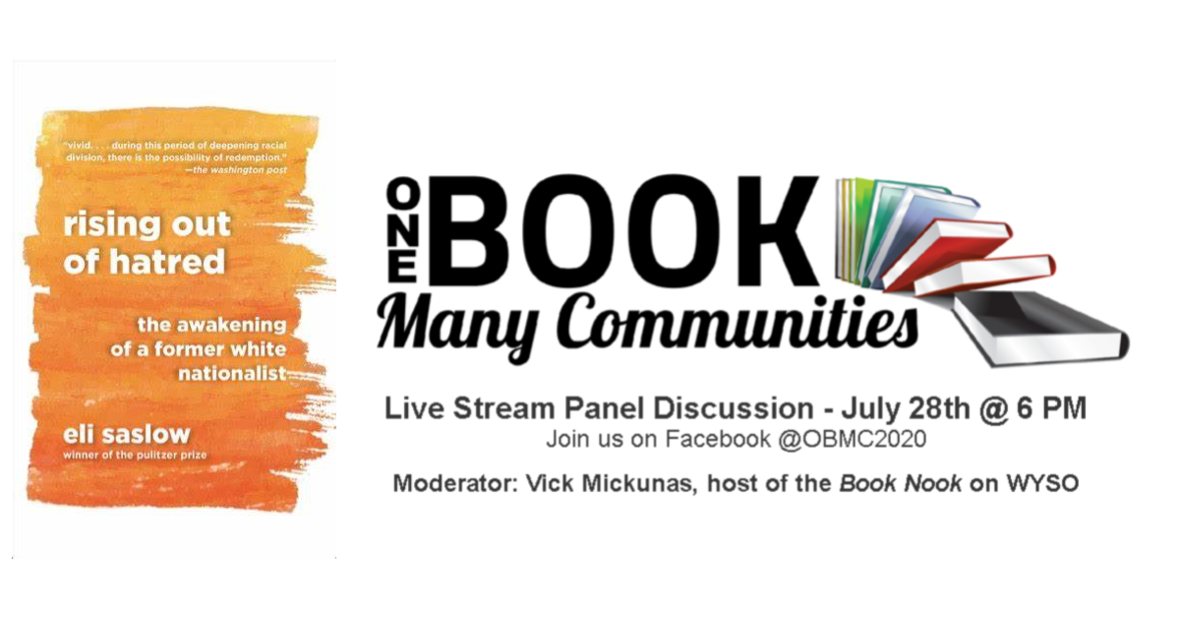table div table+table+table+table+table+table+table+table+table+table+table+table+table+table+table+table+table+table+table+table+table+table+table+table+table+table+table+table+table+table+table+table+table+table+table+table+table+table+table+table+table+table+table+table+table+table div table{width:100%;padding:0}table div table+table+table+table+table+table+table+table+table+table+table+table+table+table+table+table+table+table+table+table+table+table+table+table+table+table+table+table+table+table+table+table+table+table+table+table+table+table+table+table+table+table+table+table+table+table div table img{width:96.23%;padding:0;float:none}table div table+table+table+table+table+table+table+table+table+table+table+table+table+table+table+table+table+table+table+table+table+table+table+table+table+table+table+table+table+table+table+table+table+table+table+table+table+table+table+table+table+table+table+table+table+table div table td{width:100%;padding:0 1.88% 18px}/* styles */table div table+table+table+table+table+table+table+table+table+table+table+table+table+table+table+table+table+table+table+table+table+table+table+table+table+table+table+table+table+table+table+table+table+table+table+table+table+table+table+table+table+table+table+table+table+table+table+table div table{width:100%;padding:0}table div table+table+table+table+table+table+table+table+table+table+table+table+table+table+table+table+table+table+table+table+table+table+table+table+table+table+table+table+table+table+table+table+table+table+table+table+table+table+table+table+table+table+table+table+table+table+table+table div table img{width:96.23%;padding:0;float:none}table div table+table+table+table+table+table+table+table+table+table+table+table+table+table+table+table+table+table+table+table+table+table+table+table+table+table+table+table+table+table+table+table+table+table+table+table+table+table+table+table+table+table+table+table+table+table+table+table div table td{width:100%;padding:0 1.88% 18px}/* styles */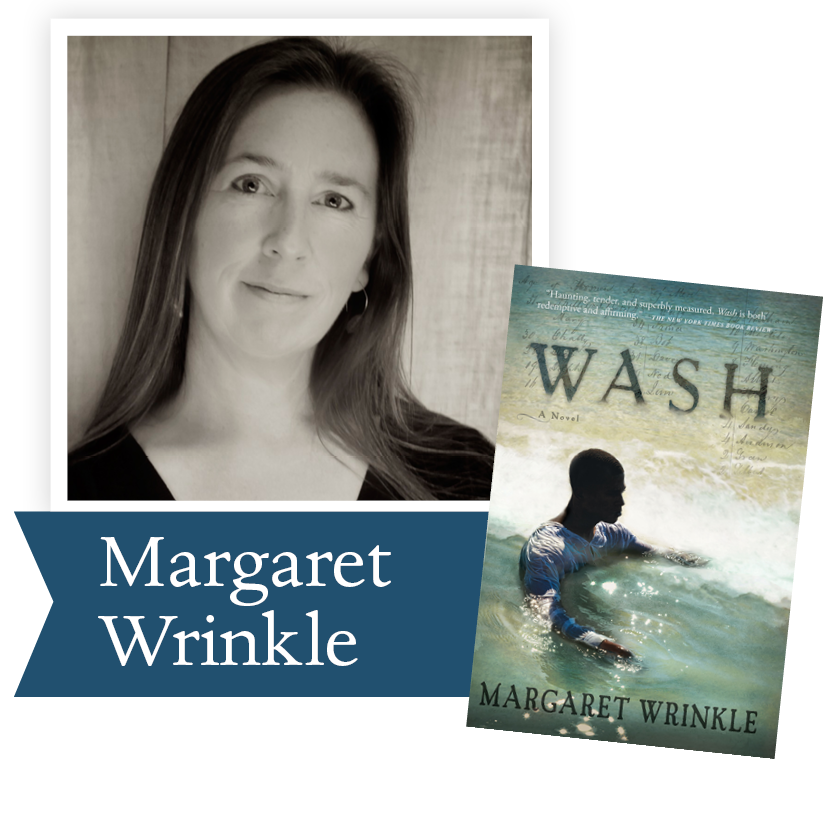Margaret Wrinkle’s debut novel Wash was the 2014 DLPP Fiction Runner-Up, and over the last six years, Margaret has generously returned to Dayton numerous times to share her insights into our past and her writing process that makes Wash an unforgettable story. Margaret takes us from Tennessee to West Africa and brings us back again as she explores the soul of the South.

“The voices of the past can't speak for themselves and must rely on the artists of the future to honor them. It's a profound responsibility and one that Margaret Wrinkle meets in her brilliant novel Wash. She shows not only the courage to submerge herself in the Stygian world of plantation slavery but also the grace and sensitivity to bring that world to life . . . Narrative roles are given to Wash, fellow slaves and his succession of masters, creating a dense, hypnotic ensemble of voices. It's from patriarchs like Wash, Ms. Wrinkle shows, that the U.S. was born.” —Sam Sacks, The Wall Street Journal

The members of the DLPP book club chose Wash so we can continue to explore the basis for the call for justice we continue to hear today. Please join us for a rich discussion of our past and our present.

Ron Rollins, recently retired Dayton Daily News Ideas and Voices Editor, will moderate the discussion.

 table div table+table+table+table+table+table+table+table+table+table+table+table+table+table+table+table+table+table+table+table+table+table+table+table+table+table+table+table+table+table+table+table+table+table+table+table+table+table+table+table+table+table+table+table+table+table+table+table+table+table div table{width:100%;padding:0}table div table+table+table+table+table+table+table+table+table+table+table+table+table+table+table+table+table+table+table+table+table+table+table+table+table+table+table+table+table+table+table+table+table+table+table+table+table+table+table+table+table+table+table+table+table+table+table+table+table+table div table img{width:96.23%;padding:0;float:none}table div table+table+table+table+table+table+table+table+table+table+table+table+table+table+table+table+table+table+table+table+table+table+table+table+table+table+table+table+table+table+table+table+table+table+table+table+table+table+table+table+table+table+table+table+table+table+table+table+table+table div table td{width:100%;padding:0 1.88% 18px}/* styles */